###### Beta coefficient

Beta coefficient, denoted as ßj, is the measure of diversifiable risks. This is defined as the degree of movement in an asset or security’s return in response to the overall market return. Symbolically, it is expressed as.

j=COVjM / Varm

Where,

j = Beta coefficient for security ‘j’.

COVjM = The covariance between the return on market portfolio and the return on security ‘j’.

VARM= Variance of the return on market portfolio.

The market return is the return on the market portfolio of all traded securities, which includes the stock market indicators such as that of Nepal Stock Exchange (NEPSE) and the beta coefficient for market portfolio is supposed to be equal to 1.0. All other betas for individual securities are viewed in relation to this value. The beta for individual securities may take on values that are either positive or negative. The beta coefficient of any security ranging above zero implies that security required return moves in the same direction as that of market return and similarly security beta coefficient ranging below zero implies that security’s required return moves in opposite direction to market. If the returns on the individual asset fluctuate by exactly the same degree as the return on the market as a whole, the beta for the security is one. Using the security’s beta coefficient (B₁), the equation for CAPM is given as follows:

E (RJ) = R + [E(Rm) – RF ] B₁.

Where,

E(Rj) = Required return on security ‘j’

RF = The risk-free rate of borrowing and lending.

E(Rm) = The expected return on market portfolio.

B₁ = Beta coefficient for security j.

In above equation, the required return on the security ‘j’ is an increasing function of beta coefficient (B₁). Higher level of non-diversifiable risk causes higher required return on the security and vice versa. The logic of the CAPM equation is that the required return on any investment is the risk-free return plus a risk adjustment factor. The risk adjustment factor is simply the risk premium required for market return, [E (Rm) – RF], multiplied by the riskiness of the individual security or assets,j

###### Capital Asset Pricing Model

Capital Assets Pricing, Model (CAPM) shows the relationship between risk and return of an asset. The CAPM postulates that the required rate of return on any asset is the total of risk-free rate plus the risk premium. The CAPM equation is stated as follows:

E(R₁) = RF + [E(RM) – RF] j

In above equation, E(R₁) refers to the expected rate of return on security j in equilibrium; RF is the risk-free rate of return; and [E(RM) – RF] B, is the risk premium on security j. The expected rate of return on security j is the rate that an investor expects to earn to compensate against the systematic risk associated with security j. It is the minimum required rate of return for an investor to induce him/her on this investment.

In CAPM context, security j’s return has two components: risk-free rate (RF) plus expected risk premium on security j. Again the expected risk premium on security j has two components: market risk premium denoted as [E(RM) – RF] multiplied by systematic risk index denoted by beta (B). The last term, [E(RM) CAPM equation is known as a security’s risk premium which is proportional to beta RF] B₁ in the and the market risk premium. It implies that the expected risk premium on security changes proportionally in the same direction with the change in market risk premium and beta. Beta is a measure of systematic risk. Generally, the risk-free rate and market risk premium is the same for all securities. However, they differ in terms of level of systematic risk denoted by security’s beta. Thus, a security with higher systematic risk will have higher beta, hence higher risk premium; and a security with lower systematic risk will have lower beta, hence lower risk premium.

2068 Q.No. 10a

Systematic risk refers to the risk which affects the whole market and therefore it cannot be reduced or diversified away. It refers to the variability in security’s return with respect to the overall market. On the other hand, unsystematic risk is the extent of variability in security’s return on account of factors which are unique to a firm. In other words, it is the variability in security’s return with respect to unique factors associated with individual firms. It can be diversified away.

Some differences between systematic and unsystematic risk are as follows:

 Basis Systematic risk Unsystematic risk Effect Systematic risk affects all securities in a market. Unsystematic risk is unique to a security or a company and does not affect all securities. Dependency This risk is dependent on political or economic factors. Higher the level of political or economic uncertainties, higher will be the systematic risk. This risk is independent of political or economic factors and unique to each individual firm. Diversifiable Systematic risk is also known as market risk and cannot be reduced by achieving diversification of investment. Unsystematic risk is also called diversifiable risk and can be reduced by diversification of investment. In other words, a portfolio with many assets has almost no unsystematic risk. Sources This arises due to imbalance in the political situation or fluctuation in the market etc. This risk arises from management inefficiency, unsuccessful planning etc.

## Numerical Problems

Question:

The market and Stock J have the following probability distributions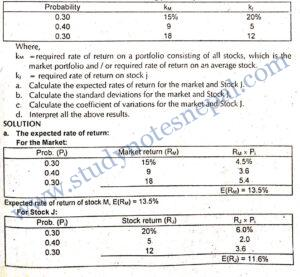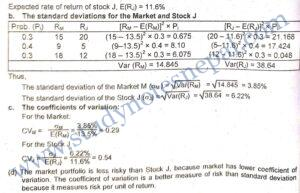Question:

Stock X and Y have the following probability distributions of expected future returns:

 Probability 0.1 0.2 0.4 0.2 0.1 X   Y – 10%   – 35% 2   0 12   20 20   25 38   45

a. Calculate the expected rate of return for stock Y. (That for X is 12%)

b. Calculate the standard deviation of expected returns for stock X. (That for stock Y is 20.35%) Now calculate the coefficient of variation for stock Y. Is it possible that most investors might regards stock Y as being less risk than stock X? Explain.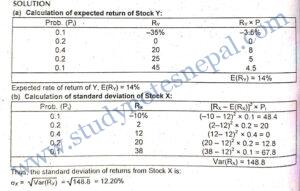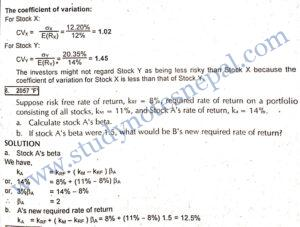Question:

Stock X and Y have the following probability distribution of expected future returns: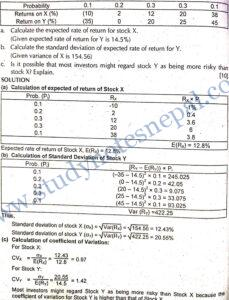Question:

Mr. Pradhan is considering investing in a security j that has the following distribution of possible one-year returns:

 Probability of occurrence 0.10 0.2 0.3 0.3 0.1 Possible return (%) (10) 0 10 20 40

Required:

(a) Calculate the expected return and standard deviation associated with the investment.

(b) If the one year market return is 14% with standard deviation of 12%, calculate the risk per unit of return for the market and stock j.

(c) Calculate the probability that stock return is zero or less. (d) Interpret the results of (a), (b) and (c) as above.

SOLUTION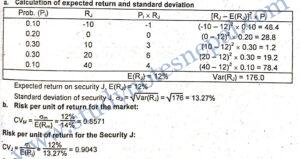c. The probability distribution of Security J’s return shows that there is 10 percent probability of negative 10 percent return and 20 percent probability that it has zero return. Thus the probability that Security J has zero or less return is the total of these probabilities, that is, 30 percent. In other words, there is a 30 percent chance that Security J offers zero or negative returns.

d. In above calculation it can be observed that for a given level of risk (that standard deviation 12%), the market portfolio has higher expected return than the Security J. Therefore its risk per unit of return as measured by coefficient of variation is lower. This implies that a market portfolio is a better alternative than Security J from both risk and return perspectives.

###### Explain the concept of portfolio risk and the capital assets pricing model.

A portfolio is a combination of wealth into two or more assets. The portfolio theory deals with forming an efficient portfolio of assets that offers higher return and minimizes the risk. It is possible for an investor that a single asset might be very risky when held in isolation but not as much risky when held in combination with other assets in the portfolio. Thus the basic idea behind forming the portfolio is to reduce investment risk. But the extent to which risk can be reduced by forming the portfolio depends on several factors.

• First, the portfolio risk depends on the riskiness of individual assets in the portfolio. – Other things remaining the same higher the level of risk associated with individual assets will be the portfolio risk. However, this is not the only complete factor.
• Besides riskiness of individual assets, portfolio risk is largely determined by the correlation between movements in returns from those assets. The correlation between assets returns may assume any values between -1 and +1. Correlation coefficient -1 implies that returns from two assets are perfectly moving into opposite directions. In this condition, the maximum risk reduction is possible by forming the portfolio. When correlation is + 1, assets returns are moving perfectly into the same direction. In this condition no risk reduction is possible. Larger risk reduction is possible by forming the portfolio when assets have correlation coefficient closer to -1. Thus, correlation also does matter for portfolio risk. This is well explained by Markowitz diversification, which calls for combining assets with less than perfect positive correlation coefficient to reduce portfolio risk. The Markowitz diversification also asserts that correlation is exactly -1. and if optimal allocation of wealth into the assets in the portfolio can be attained, the portfolio risk can even be reduced to zero.
• Reducing portfolio risk to zero can be attained only if assets returns are perfectly negatively correlated. But in practice, perfect negative or perfect positive correlation is hardly observed. Thus, by forming the portfolio risk can be reduced but not completely eliminated. According to simple diversification, if we go on adding more assets in the portfolio, the risk can be reduced. The risk which can be eliminated by forming an efficient portfolio is the unsystematic risk, not the systematic risk. This notion is well explained by the Capital Asset Pricing Model (CAPM).

Capital Assets Pricing Model (CAPM) shows the relationship between risk and return of an asset.. The CAPM postulates that the required rate of return on any asset is the total of risk-free rate plus the risk premium. The CAPM equation is stated as follows:

E(R) = R + [E(RM) – RF] B₁

In the above equation, E(R) refers to the expected rate of return on security j in equilibrium; RF is the risk-free rate of return; and [E(RM) – RF] B, is the risk premium on security j. The expected rate of return on security j is the rate that an investor expects to earn to compensate against the systematic risk associated with security. j. It is the minimum required rate of return for an investor to induce him/her on this investment.

In CAPM context, security j’s return has two components: risk-free rate (RF) plus expected risk premium on security j. Again the expected risk premium on security j has two components: market risk premium denoted as [E(RM) – RF] multiplied by

systematic risk index denoted by beta (3₁). The last term, [E(RM) – RF) B, in the CAPM equation is known as a security’s risk premium which is proportional to beta and the market risk premium. It implies that expected risk premium on security j changes proportionally in the same direction with the change in market risk premium and beta. Beta is a measure of systematic risk. Generally, the risk-free rate and market risk premium is the same for all securities. However, they differ in terms of level of systematic risk denoted by security’s beta. Thus, a security with higher systematic risk. will have higher beta, hence higher risk premium; and a security with lower systematic risk will have lower beta, hence lower risk premium.

Question:

Stock X and Y have the following probability distributions of expected future returns:

 Probability 0.1 0.2 0.4 0.2 0.1 X   Y – 10%   – 35% 2   0 12   20 20   25 38   45
1. Calculate the expected rate of return for stock Y. (That for X is 12%)
2. Calculate the standard deviation of expected returns for stock X. (That for stock Y is 20.35%) Now calculate the coefficient of variation for stock Y. Is it possible that most investors might regard stock Y as being less risky than stock X? Explain.